# Stat-Ease Blog

## Improving Your Predictive Model via a Response Transformation

posted by Shari Kraber on Jan. 5, 2022

A good predictive model must exhibit overall significance and, ideally, insignificant lack of fit plus high adjusted and predicted R-squared values. Furthermore, to ensure statistical validity (e.g., normality, constant variance) the model’s residuals must pass a series of diagnostic tests (fortunately made easy by Stat-Ease software):

• Normal plot of residuals illustrates a relatively straight line. If you can cover the residuals with a fat pencil, no worries, but watch out for a pronounced S-shaped curve such as Figure 1 exhibits.
• Residuals-versus-predicted plot has points scattered randomly, i.e., demonstrating a constant variance from left to right. Beware of a “megaphone” shape as seen in Figure 2.
• Residuals-versus-run plot exhibiting no trends, shifts or outliers (points outside the red lines such as seen in Figure 3).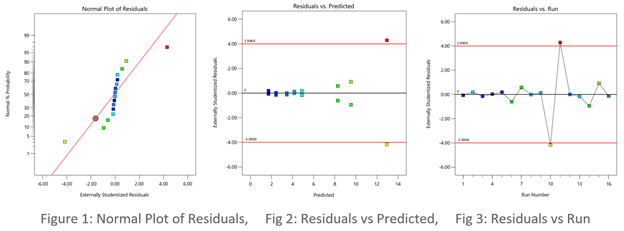When diagnostic plots of residuals do not pass the tests, the first thing you should consider for a remedy is a response transformation, e.g., rescaling the data via a natural log (again made easy by Stat-Ease software). Then re-fit the model and re-check the diagnostic plots. Often you will see improvements in both the statistics and the plots of residuals.

The Box-Cox plot (see Figure 4) makes the choice of transformation very simple. Based on the fitted model, this diagnostic displays a comparable measure of residuals against a range of power transformations, e.g., taking the inverse of all your responses (lambda -1), or squaring them all (lambda 2). Obviously, the lower the residuals the better. However, only go for a transformation if your current responses at the power of 1 (the blue line), fall outside the red-lined confidence interval, such as Figure 4 display. Then, rather than going to the exact-optimal power (green line), select one that will be simpler (and easier to explain)--the log transformation in this case (conveniently recommended by Stat-Ease software).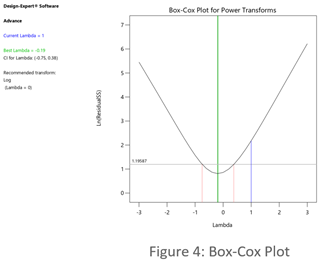See the improvement made by the log transformation in the diagnostics (Figures 5, 6 and 7). All good!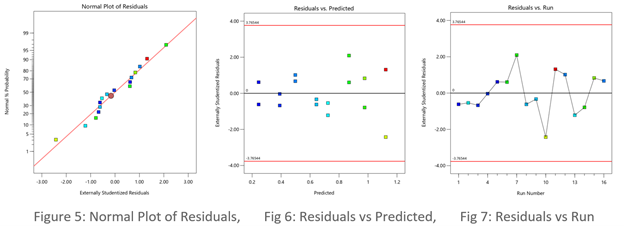In conclusion, before pressing ahead with any model (or abandoning it), always check the residual diagnostics. If you see any strange patterns, consider a response transformation, particularly if advised to do so by the Box-Cox plot. Then confirm the diagnostics after re-fitting the model.

For more details on diagnostics and transformations see How to Use Graphs to Diagnose and Deal with Bad Experimental Data.

~ Shari Kraber, [email protected]

## R-Squared Mysteries Solved

posted by Shari Kraber on Nov. 9, 2021

Design of experiments (DOE) and the resulting data analysis yields a prediction equation plus a variety of summary statistics. A set of R-squared values are commonly used to determine the goodness of model fit. In this blog, I peel back the raw versus adjusted versus predicted R-squared and explain how each can be interpreted, along with the relationships between them. The calculations of these values can be easily found online, so I won’t spend time on that, focusing instead on practical interpretations and tips.

Raw R-squared measures the fraction of variation explained by the fitted predictive model. This is a good statistic for comparing models that all have the same number of terms (like comparing models consisting of A+B versus A+C). The downfall of this statistic is that it can be artificially increased simply by adding more terms to the model, even ones that are not statistically significant. For example, notice in Table 1 from an optimization experiment how R-squared increases as the model steps up in order from linear to two-factor interaction (2FI), quadratic and, finally, cubic (disregarding it being aliased).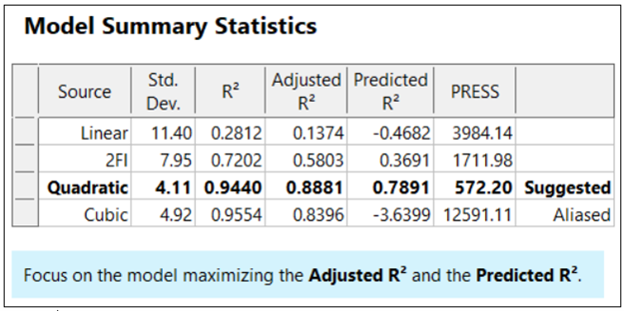The “adjusted” R-squared statistic corrects this ‘inflation’ by penalizing terms that do not add statistical value. Thus, the adjusted R-squared statistic generally levels off (at 0.8881 in this case) and then begins to decrease at some point as seen in Table 1 for the cubic model (0.8396). The adjusted R-squared value cannot be inflated by including too many model terms. Therefore, you should report this measure of model fit, not the raw R-squared.

The “predicted” R-squared is most rigorous for assessing model fit, so much so that it often starts off negative at the linear order, as it does for the example in Table 1 (-0.4682). As you can see, this statistic improves greatly as significant terms are added to the model, and quickly decreases once non-significant terms are added, e.g., going negative again at cubic. If predicted R-squared goes negative, the model becomes worse than nothing, that is, simply taking the average of the data (a “mean” model)—that is not good!

Figures 1 illustrates how the predicted R-squared peaks at the quadratic model for the example. Once a model emerges at the highest adjusted and/or predicted R-squared, consider taking out any insignificant terms—best done with the aid of a computerized reduction algorithm. This often produces a big increase in the predicted R-squared.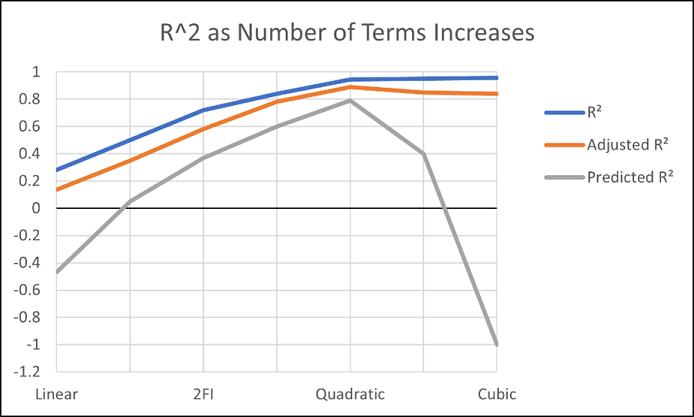Conclusion

The goal of modeling data is to correctly identify the terms that explain the relationship between the factors and the response. Use the adjusted R-squared and predicted R-squared values to evaluate how well the model is working, not the raw R-squared.

PS: You’ve likely been reading this expecting to find recommended adjusted and predicted R-squared values. I will not be providing this. Higher values indicate that more variation in the data or in predictions is explained by the model. How you use the model dictates the threshold that is acceptable to you. If the DOE goal is screening, low values can be acceptable. Remember that low R-squared values do not invalidate significant p-values. In other words, if you discover factors that have strong effects on the response, that is positive information, even if the model doesn’t predict well. A low predicted R-squared means that there is more unexplained variation in the system, and you have more work to do!

## Why it pays to be skeptical of three-factor-interaction effects

posted by Mark Anderson on Sept. 29, 2021

Quite often, when providing statistical help for Stat-Ease software users, our consulting team sees an over-selection of effects from two-level factorial experiments. Generally, the line gets crossed when picking three-factor interactions (3FI), as I documented in the lead article for the June 2007 Stat-Teaser. In this case, the experimenter picked all the estimable effects when only one main effect (factor B) really stood out on the Pareto plot. Check it out!

In my experience, the true 3FIs emerge only when one of the variables is categorical with a very strong contrast. For example, early in my career as an R&D chemical engineer with General Mills, I developed a continuous process for hydrogenating a vegetable oil. By cranking up the pressure and temperature and using an expensive, noble-metal catalyst (palladium on a fixed bed of carbon), this new approach increased the throughput tremendously over the old batch process, which deployed powered nickel to facilitate the reaction. When setting up my factorial experiment, our engineering team knew better than to make the type of reactor one of the inputs, because being so different, this would generate many complications of time-temperature interactions differing from on process to the other. In cases like this, you are far better off doing separate optimizations and then seeing which process wins out in the end. (Unfortunately for me, I lost this battle due to the color bodies in the oil poisoning my costly catalyst.)

A response must really behave radically to require a 3FI for modeling as illustrated hypothetically in Figures 1 versus 2 for two factors—catalyst level (B) and temperature (D)—as a function of a third variable (E)—the atmosphere in the reactor.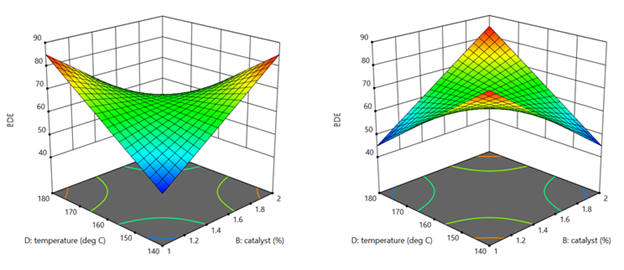Figures 1 & 2: 3FI (BDE) surface with atmosphere of nitrogen vs air (Factor E at low & high levels)

These surfaces ‘flip-flop’ completely like a bird in flight. Although factor E being categorical does lead to a strong possibility of complex behavior from this experiment, the dramatic shift caused by it changing from one level to the other would be highly unusual by my reckoning.

It turns out that there is a middle ground with factorial models that obviates the need for third-order terms: Multiple two-factor interactions (2FIs) that share common factors. The actual predictive model, derived from a case study we present in our Modern DOE for Process Optimization workshop, is:

Yield = 63.38 + 9.88*B + 5.25*D − 3.00*E + 6.75*BD − 5.38*DE

Notice that this equation features two 2FIs, BD and DE, that share a common factor (D). This causes the dynamic behavior shown in Figures 3 and 4 without the need for 3FI terms.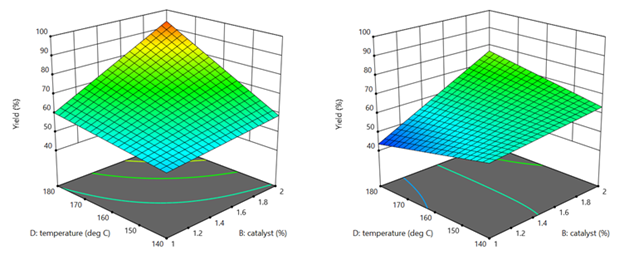Figure 3 & 4: 2FI surface (BD) for atmosphere of nitrogen vs air (Factor E at low & high levels)

This simpler model sufficed to see that it would be best to blanket the batch reactor with nitrogen, that is, do not leave the hatch open to the air—a happy ending.

Conclusion

If it seems from graphical or other methods of effect selection that 3FI(s) should be included in your factorial model, be on guard for:

• Over-selection of effects (my first case)
• The need for a transformation (such as log): Be sure to check the Box-Cox plot (always!).
• Outlier(s) in your response (look over the diagnostic plots, especially the residual versus run).
• A combination of these and other issues—ask [email protected] for guidance if you use Stat-Ease software (send in the file, please).

I never say “never”, so if you really do find a 3FI, get back to me directly.

-Mark ([email protected])

## Christmas Trees on my Effects Plot?

posted by Shari Kraber on Dec. 3, 2020

As a Stat-Ease statistical consultant, I am often asked, “What are the green triangles (Christmas trees) on my half-normal plot of effects?”

Factorial design analysis utilizes a half-normal probability plot to identify the largest effects to model, leaving the remaining small effects to provide an error estimate. Green triangles appear when you have included replicates in the design, often at the center point. Unlike the orange and blue squares, which are factor effect estimates, the green triangles are noise effect estimates, or “pure error”. The green triangles represent the amount of variation in the replicates, with the number of triangles corresponding to the degrees of freedom (df) from the replicates. For example, five center points would have four df, hence four triangles appear. The triangles are positioned within the factor effects to reflect the relative size of the noise effect. Ideally, the green triangles will land in the lower left corner, near zero. (See Figure 1). In this position, they are combined with the smallest (insignificant) effects and help position the red line. Factor effects that jump off that line to the right are most likely significant. Consider the triangles as an extra piece of information that increases your ability to find significant effects.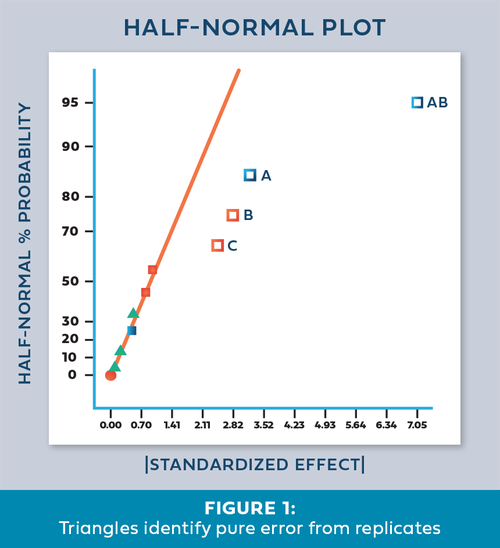Once in a while we encounter an effects plot that looks like Figure 2. “What does it mean when the green triangles are out of place - on the upper right side instead of the lower left?”

This indicates that the variation between the replicates is greater than the largest factor effects! Since this error is part of the normal process variation, you cannot say that any of the factor effects are statistically significant. At this point you should first check the replicate data to make sure it was both measured and recorded correctly. Then, carefully consider the sources of process variation to determine how the variation could be reduced. For a situation like this, either reduce the noise or increase the factor ranges. This generates larger signals that allow you to discover the significant effects.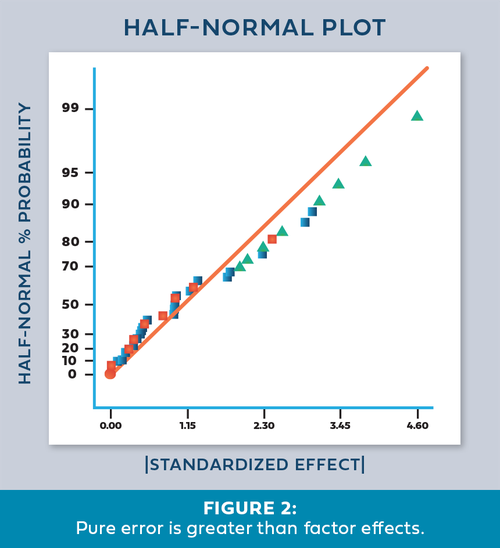- Shari Kraber

For statistical details, read “Use of Replication in Almost Unreplicated Factorials” by Larntz and Whitcomb.

## Greg's DOE Adventure - Factorial Design, Part 2

posted by Greg on April 15, 2020

[Disclaimer: I’m not a statistician. Nor do I want you to think that I am. I am a marketing guy (with a few years of biochemistry lab experience) learning the basics of statistics, design of experiments (DOE) in particular. This series of blog posts is meant to be a light-hearted chronicle of my travels in the land of DOE, not be a text book for statistics. So please, take it as it is meant to be taken. Thanks!]

#### Keep your experiment planned, but random

When I wrote my introduction to factorial design (Greg’s DOE Adventure - Factorial Design, Part 1), there were a couple of points that I left out. I’ll amend that post here to talk about making sure your experiment is planned out yet random.

Wait. What?

You’ll see. Let me explain.

Getting organized

During the initial phase of an experiment, you should make sure that it is well planned out. First, think about the factors that affect the outcome of your experiment. You want to create a list that’s as all-encompassing as possible. Anything that may change the outcome, put on your list. Then pare it down into the ones that you know are going to be the biggest contributors.

Once you have done that, you can set the levels at which to run each factor. You want the low and high levels to be as far apart as possible. Not too low that you won’t see an effect (if your experiment is cooking something, don’t set the temperature so low that nothing happens). Not too high that it’s dangerous (as in cooking, you don’t want to burn your product).

Finally, you want to make sure your experiment is balanced when it comes to the factors in your experiment. Taking the cooking example above a little further, suppose you have three factors you are testing: time, temperature, and ingredient quality. Let’s also say that you are testing at two different levels: low and high (symbolized by minus and plus signs, respectively). We can write this out in a table: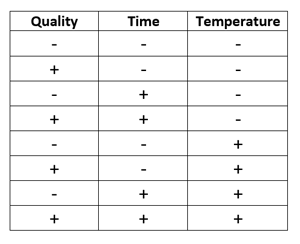This table contains all the possible combinations of the three factors. It’s called an ‘orthogonal array’ because it’s balanced. Each column has the same number of pluses and minuses (4 in this case). This balance in the array allows all factors to be uncorrelated and independent from each other.

With these steps, you have ensured that your experiment is well planned out and balanced when looking at your factors.

Always randomize

At the start of this post, I said that an experiment should be planned out, yet random. Well we have the planned-out part, now let’s get into the random part.

In any experimentation, influence from external sources (variables you are not studying) should be kept to a minimum. One way to do this is randomizing your runs.

As an example, let’s look at the table above with the cooking example. Let’s say that it represents the order of how the experiment was run. So, all the low temperature runs were made together and then all the high ones together. This makes sense, right? Perform all the runs at one temperature before adjusting up to the next setting.

The problem is, what if there is an issue with your oven that causes the temperature to fluctuate more, early in the experiment and less later. This time-related issue introduces variation (bias) into your results that you didn’t know about.

To reduce the influence of this variable, randomize your run order. It may take more time adjusting your oven for every run, but it will remove that unwanted variation.

Temperature is a popular example to illustrate randomization. But this can be said of any factor that may have time-related problems. It could be warm-up time on a machine or the physical tiring of an operator. Randomization is used to guard against bias as much as you can when running an experiment.

Conclusions

Hopefully, you see now why I said to keep your experiments planned but random. It sounds like an oxymoron, but it’s not. Not in the way I’m talking about it here!